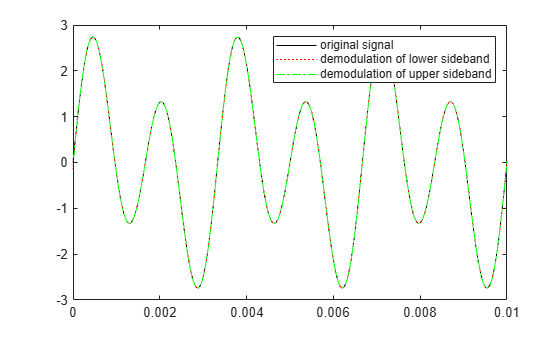# ssbdemod

Single sideband amplitude demodulation

## Syntax

```z = ssbdemod(y,Fc,Fs) z = ssbdemod(y,Fc,Fs,ini_phase) z = ssbdemod(y,Fc,Fs,ini_phase,num,den) ```

## Description

### For All Syntaxes

`z = ssbdemod(y,Fc,Fs)` demodulates the single sideband amplitude modulated signal `y` from the carrier signal having frequency `Fc` (Hz). The carrier signal and `y` have sampling rate `Fs` (Hz). The modulated signal has zero initial phase, and can be an upper- or lower-sideband signal. The demodulation process uses the lowpass filter specified by `[num,den] = butter(5,Fc*2/Fs)`.

Note

The `Fc` and `Fs` arguments must satisfy `Fs` > 2(`Fc` + `BW`), where `BW` is the bandwidth of the original signal that was modulated.

`z = ssbdemod(y,Fc,Fs,ini_phase)` specifies the initial phase of the modulated signal in radians.

`z = ssbdemod(y,Fc,Fs,ini_phase,num,den)` specifies the numerator and denominator of the lowpass filter used in the demodulation.

## Examples

collapse all

Define the sampling frequency and original signal.

```fs = 270000; t = (0:1/fs:0.01)'; signal = sin(2*pi*300.*t)+2*sin(2*pi*600.*t);```

Convert the original signal to upper-sideband and lower-sideband modulated signals using `ssbmod`. Use a cutoff frequency of `12000` and an initial phase of `0`.

```fc = 12000; initialPhase = 0; lowerSidebandSignal = ssbmod(signal,fc,fs,initialPhase); upperSidebandSignal = ssbmod(signal,fc,fs,initialPhase,'upper');```

Demodulate the lower and upper sideband signals.

```s1 = ssbdemod(lowerSidebandSignal,fc,fs); s2 = ssbdemod(upperSidebandSignal,fc,fs);```

Compare processed signals with original and verify reconstruction.

```plot(t,signal,'k',t,s1,'r:',t,s2,'g-.'); legend('Original Signal','Demodulation of Lower Sideband','Demodulation of Upper Sideband');```